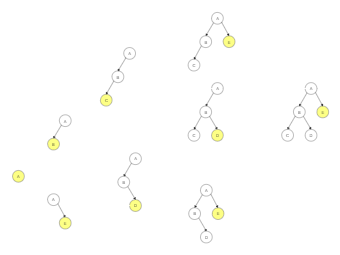Template Community / Math Diagram

# Math Diagram

Share
Duplicate8
0
Report
Publish time：07-16-2021
A tree is a hierarchical data structure defined as a collection of nodes. Nodes represent value, and nodes are connected by edges. A tree has the following properties: The tree has one node called root, each node has one parent only but can have multiple children, each node is connected to its children via edges. In a Binary tree, every node can have at most two children, left and right. In the diagram below, C & D have left children, and E is the right child. It should be noted here that the math tree structure is used in predictive modeling. It is usually called a Decision tree. Each internal node represents a test or condition on a predictive variable in the math decision tree, and the edge gives various possible answers to this test.Trestrals ZPost
Recommended Templates